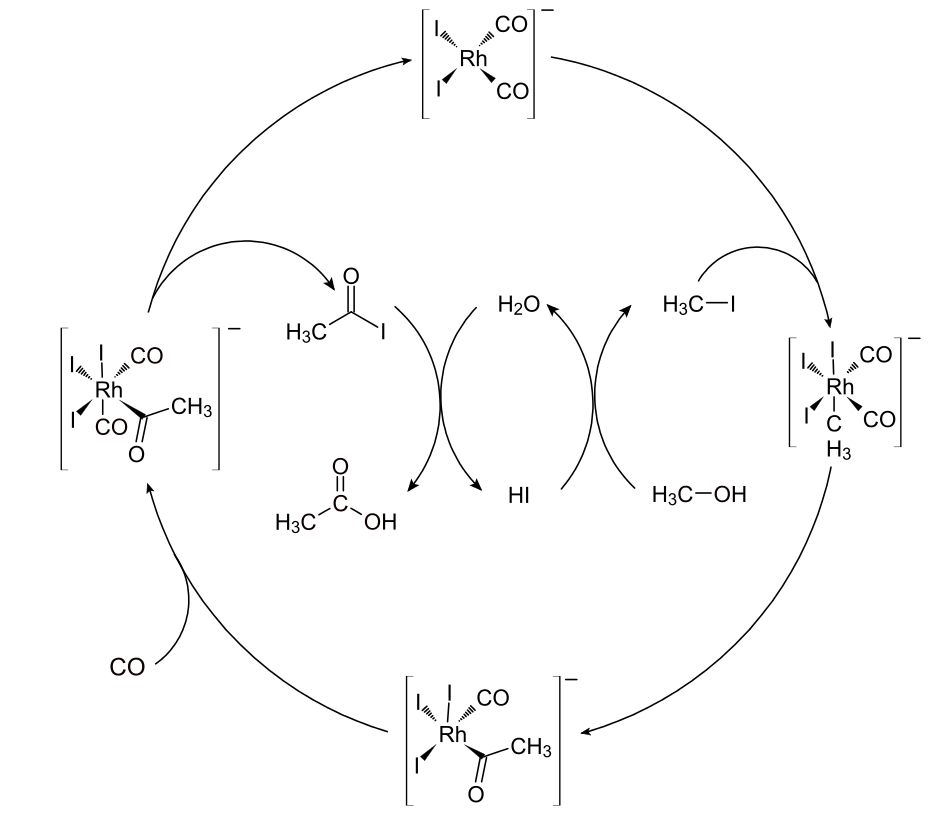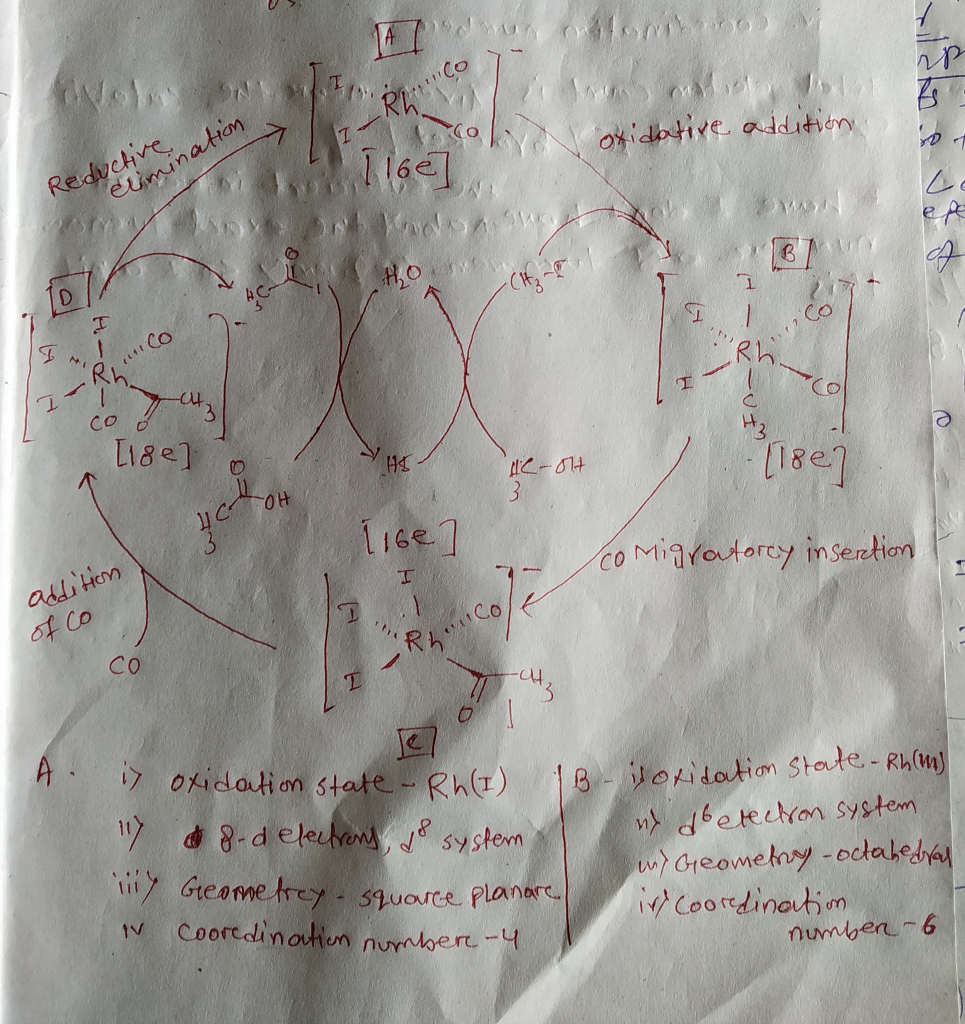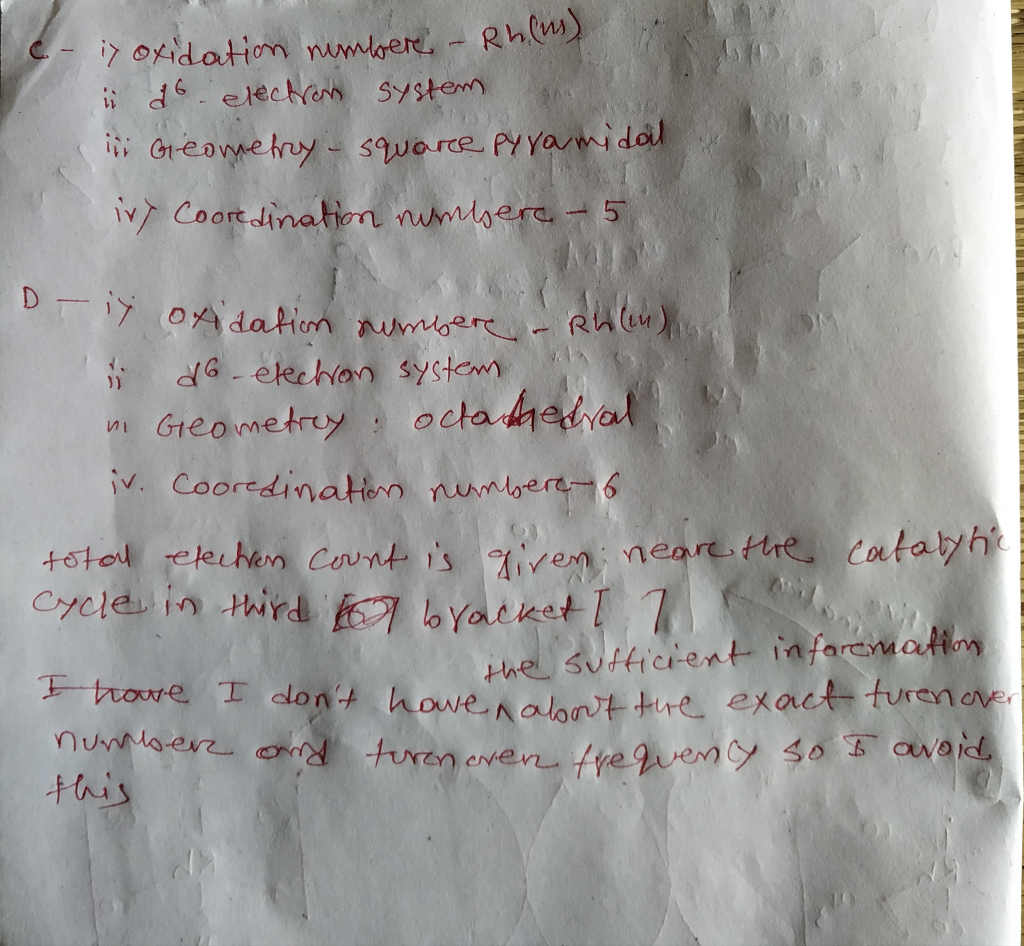Homework Help Question & Answers

For each complex in the Monsanto Acetic Acid Process, indicate the following: 1. Name of step 2. ...

For each complex in the Monsanto Acetic Acid Process, indicate the following:

1. Name of step

2. oxidation state of the metal

3. d electron count

4. total electron count

5. turnover number

6. turnover frequency

7. geometry

8. coordination numberCO Rh CO H3C-I H20 CO Rh H3C CO h CH3 CO Нас-он HI CO COAdd Answer of: For each complex in the Monsanto Acetic Acid Process, indicate the following: 1. Name of step 2. ...
More Homework Help Questions Additional questions in this topic.

• Question 4. a.) Calculate the pH of a solution initially 0.1 M in acetic acid and 0.02 M in sodium acetate. The pKa for acetic acid is 4.76. b) Calculate the pH of the buffer if you add 10 ml of 0.1M...

Need Online Homework Help?

Get FREE EXPERT Answers
WITHIN MINUTES
Related Questions